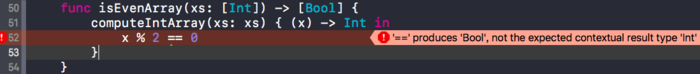# 高阶函数---swift中的泛型介绍(一步步实现Map函数)# 泛型介绍

``````func incrementArray(xs: [Int]) -> [Int] {
var result: [Int] = []
for x in xs {
result.append(x + 1)
}
return result
}
``````

``````func doubleArray(xs: [Int]) -> [Int] {
var result: [Int] = []
for x in xs {
result.append(x * 2)
}
return result
}
``````

``````func computeIntArray(xs: [Int], transform: ((Int) -> Int) ) -> [Int] {
var result: [Int] = []
for x in xs {
result.append(transform(x))
}
return result
}
``````

``````func doubleArrany2(xs: [Int]) -> [Int] {
return computeIntArray(xs: xs, transform: { (x) -> Int in
x * 2
})
}
``````

``````func isEvenArray(xs: [Int]) -> [Bool] {
computeIntArray(xs: xs) { (x) -> Int in
x % 2 == 0
}
}
````````````func computeBoolArray(xs: [Int], transform2: (Int) -> Bool) -> [Bool] {
var result: [Bool] = []
for x in xs {
result.append(transform2(x))
}
return result
}
``````

``````func genericComputeArray1<T>(xs: [Int], transform: (Int) -> T) -> [T] {
var result: [T] = []
for x in xs {
result.append(transform(x))
}
return result
}
``````

``````func map<Element, T>(xs: [Element], transform: (Element) -> T) -> [T] {
var result: [T] = []
for element in xs {
result.append(transform(element))
}
return result
}
``````

``````func genericComputeArray<T>(xs: [Int], transform: (Int) -> T) -> [T] {
return map(xs: xs, transform: transform)
}
``````

``````extension Array {
func map<T>(transform: (Element) -> T) -> [T] {
var result: [T] = []
for x in self {
result.append(transform(x))
}
return result
}
}
``````

``````func genericComputeArray<T>(xs: [Int], transform: (Int) -> T) -> [T] {
return xs.map(transform: transform)
}
``````

# 顶层函数 和 类型扩展

`Swift`标准库最初的版本中, 顶层函数仍然是无处不在的, 但伴随`Swift2.0`的诞生, 这种模式被彻底从标准库中移除了. 随着`协议扩展(protocol extension)`, 当前第三方开发者有了一个强有力的工具来定义他们自己的扩展–现在我们不仅仅可以再`Array`这样的具体类型上进行定义, 还可以在`Sequence Type`一样的协议上来定义扩展.

# 下一篇